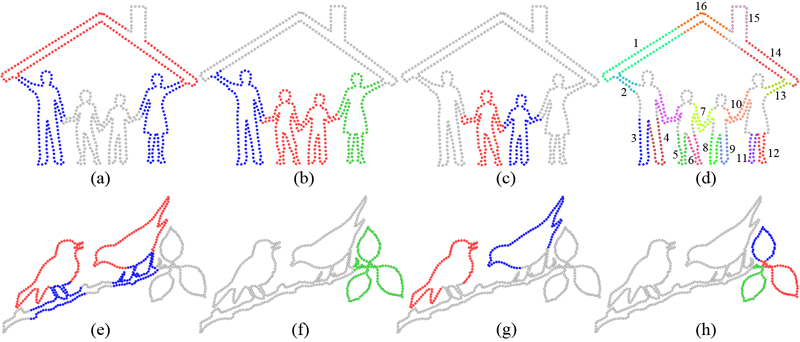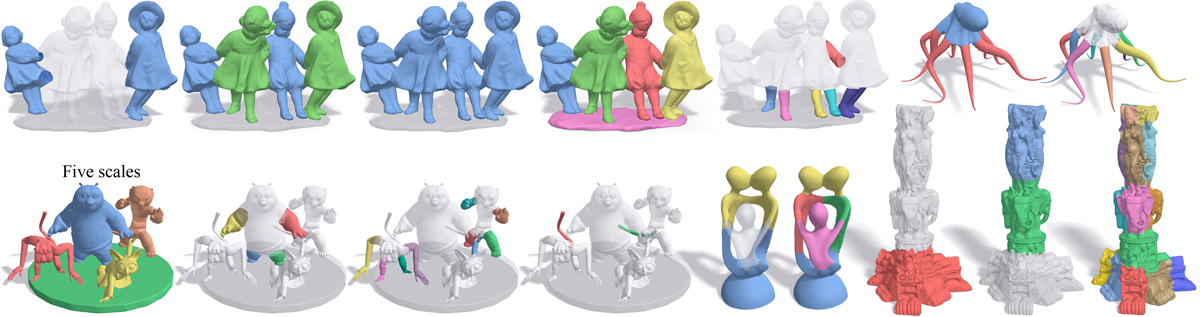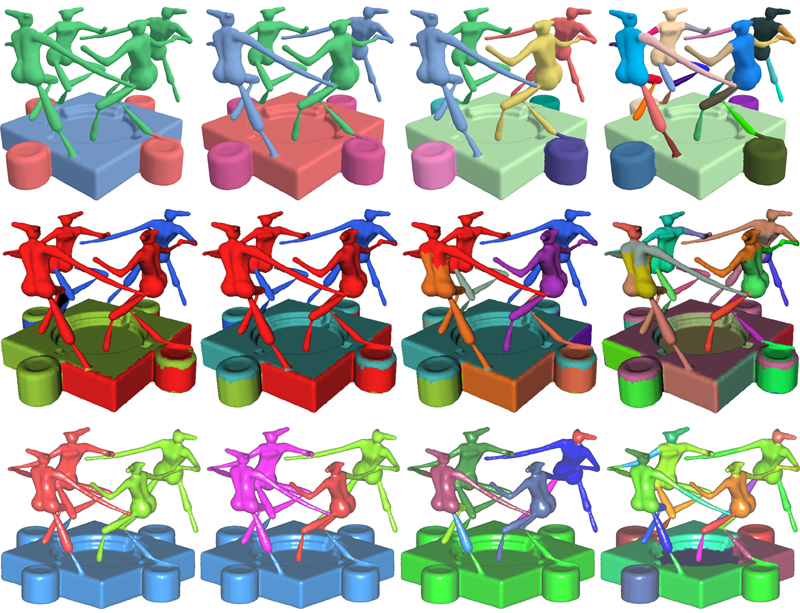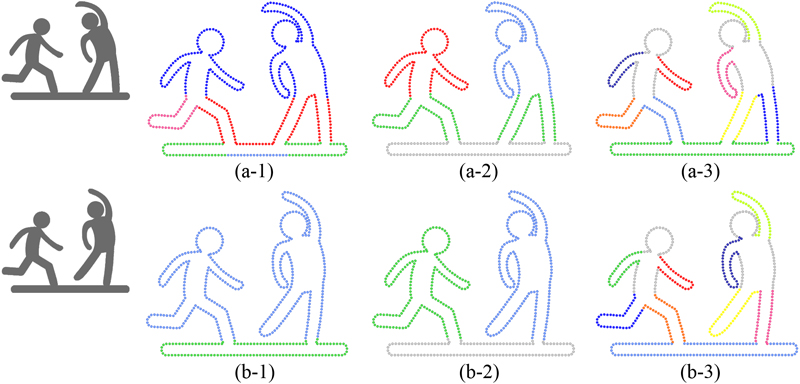Multi-Scale Partial Intrinsic Symmetry Detection

Kai Xu1,2,  Hao Zhang3,  Wei Jiang1Ramsay Dyer4Zhiquan Cheng1Ligang Liu5Baoquan Chen2

1National University of Defense Technology,     2Shenzhen VisuCA Key Lab/SIAT,
3
Simon Fraser University,     4INRIA, Geometrica,     5University of Science and Technology of China

ACM Transactions on Graphics (SIGGRAPH Asia 2012), 31(6)Figure 1: Multi-scale partial intrinsic symmetry detection: five symmetry scales (large to small) are detected. Each symmetric region is shown in uniform color. Note the detection of inter- and intra-object symmetries, as well as cylindrical symmetry of the limbs.

Abstract
We present an algorithm for multi-scale partial intrinsic symmetry detection over 2D and 3D shapes, where the scale of a symmetric region is defined by intrinsic distances between symmetric points over the region. To identify prominent symmetric regions which overlap and vary in form and scale, we decouple scale extraction and symmetry extraction by performing two levels of clustering. First, significant symmetry scales are identified by clustering sample point pairs from an input shape. Since different point pairs can share a common point, shape regions covered by points in different scale clusters can overlap. We introduce the symmetry scale matrix (SSM), where each entry estimates the likelihood two point pairs belong to symmetries at the same scale. The pair-to-pair symmetry affinity is computed based on a pair signature which encodes scales. We perform spectral clustering using the SSM to obtain the scale clsters. Then for all points belonging to the same scale cluster, we perform the second-level spectral clustering, based on a novel point-to-point symmetry affinity measure, to extract partial symmetries at that scale. We demonstrate our algorithm on complex shapes possessing rich symmetries at multiple scales.

PaperPDF, 16MB

SlidesPPTX, 16MB

ImagesFigure 2: Outline of our multi-scale symmetry detection algorithm. After a voting step which identifies a set of (sufficiently) symmetric sample point pairs (a), we perform clustering of these point pairs based on a scale-aware affinity matrix (the SSM) to determine scale clusters. In each scale cluster, we perform the second-level clustering of sample points to detect symmetries at that scale (c-d).Figure 3: Symmetry detection on 2D shapes sorted by scale. Observe the inter- and intra-object symmetries detected at multiple scales, even down to the small scales of the limbs in (d).Figure 4: A gallery of 3D symmetry detection results sorted by scale. From left to right and top-down: Children, Octopus, Kung Fu Panda, IndoLady, and Thai Statue. The last two models show raw clustering results. The IndoLady and Thai Statue models were chosen to demonstrate the performance of our algorithm on models which are not compositions of articulated characters. For the children model, not all arms or legs are detected in the last shown scale due to scale discrepancies and some parts fused with the body. For the IndoLady, our method does not return all perceived symmetries, e.g., the self-symmetries of the individual limbs. To save space, the first image for the Kung Fu Panda contains the first five scales, each revealing a self-symmetry of the four characters and the base.Figure 5: Comparison between our multi-scale results (a) to the symmetry detection results of [Lipman et al. 2010] (b) and [Xu et al. 2009] (c), which both provide only a single coverage of the shape. Our method detects overlapping symmetries and these results combine those from the other two methods.Figure 6: Plots of the top two eigenvectors of Global Point Signature (a-b), Heat Kernel Signature (c-d), and our partial intrinsic SCM in two scales (e-h). The partial intrinsic symmetries are more clearly revealed in (e-h).Figure 7: Comparison between our symmetry-driven hierarchical segmentation scheme (top row) and hierarchical segmentation based on primitive fitting [Attene et al. 2006] (middle row) and normalized cut [Golovinskiy and Funkhouser 2008] (bottom row). Each column shows the same segmentation count. It is evident that our results conform better to the shape semantics.Figure 8: Limitation to the use of intrinsic distances (a 2D case). Having one foot of the right figure planted into the base (a) or disconnected from the base (b) has a drastic effect on the multi-scale symmetries detected, since the distances changed drastically.

Thanks
We would first like to thank the anonymous reviewers for their valuable feedback. Thanks also go to Daniel Cohen-Or for fruitful discussions on the paper. Part of the 3D models in this paper is from the shape repositories of AIM@SHAPE and Stanford. This work is supported in part by grants from NSFC (61202333, 61232011, 61161160567, 61025012, 61103084, and 61070071), NSERC (No. 611370), National 863 Program (2011AA010503), Shenzhen Science and Innovation Program (CXB201104220029A, JC201005270329A), the 973 National Basic Research Program of China (2011CB302400).

Data
We provide the datasets (including both 2D and 3D shapes) used in this paper:

Dataset (ZIP, 53MB)

Bibtex
@article {xu_siga12,
title = {Multi-Scale Partial Intrinsic Symmetry Detection},
author = {Kai Xu and Hao Zhang and Wei Jiang and Ramsay Dyer and Zhiquan Cheng and Ligang Liu and Baoquan Chen}
journal = {ACM Transactions on Graphics, (Proc. of SIGGRAPH Asia 2012)},
volume = {31},
number = {6},

pages = {181:1--181:11},
year = {2012}
}